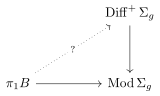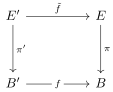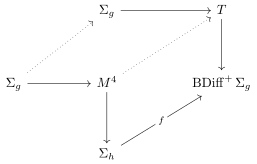# Part 2: Surface bundles, mapping class groups, moduli spaces, and cohomology

29th Workshop in Geometric Topology, Oregon State University, June 29, 2012

• Talk about the theory surface bundles.
• This talk: not so much related to his personal work!
• Will revisit

Throughout: $$\Sigma_g$$ will be a connected oriented $$g\geq 2$$ surface, will look at $$\Sigma_g{\hbox{-}}$$ bundles given by \begin{align*} \Sigma_g \to E \xrightarrow{\pi} B \end{align*}

(Fiber bundle where the base and total space will usually be compact manifolds, fiber is a surface)

For any $$f\in \operatorname{Diff}(\Sigma_g)$$, take cylinder on $$\Sigma_g$$ and glue ends via $$f$$ to obtain a 3-manifold and the surface bundle \begin{align*} \Sigma_g \to M_f^3 \xrightarrow{\pi} S^1 \end{align*}

Every 3-manifold that fibers over the circle is of this form! Why? Can remove a point from $$S^1$$ to get a trivial bundle.

Another way to build bundles: take a representation $$\rho: \pi_1 B \to \operatorname{Diff}(\Sigma_g)$$, let \begin{align*}E = \tilde B \times\Sigma_g / (x,y) \sim (g.x, \rho(g)(y))\end{align*} (standard construction) where $$\tilde B$$ is the universal cover of $$B$$.

Generalizes previous example: take real line cross surface and mod out by deck transformations.

Such bundles are called flat $$\Sigma_g$$ bundles, exactly those which admit flat connections.

Note on connections: there’s a map $$TE \xrightarrow{\pi} TB$$, can look at $$\ker \pi$$ which is a 2-plane bundle over $$E$$. Take the kernel of $$E \xrightarrow{f} B$$ is obtain a “vertical” 2-plane bundle, horizontal is not well-defined and so is a choice of a 2-plane field. Flat means curvature vanishes is equivalent to Frobenius integrability theorem – the 2-plane is integrable. So curvature measures how integrable the 2-plane field is, rephrases “when is a 2-plane tangent to a foliation”.

One organizing problem: classify surface bundles over a fixed closed base manifold up to

• Bundle isomorphism
• Fiber-wise homeomorphism, but doesn’t actually permute any fibers
• Covering the identity map
• Homeomorphism
• Diffeomorphism
• Homotopy-equivalence
• Fiber-wise Homeomorphism
• Homeomorphism that takes fiber to fiber
• Symplectomorphism
• Biholomorphism
• Total spaces are complex manifolds

B.F. proved algorithmic computability for bundle isomorphisms, but homeomorphisms and even fiberwise homeomorphisms are generally unsolvable.

Why study $$\Sigma_g$$ bundles?

1. The simplest nonlinear bundle theory (i.e. structure group is not just $$\operatorname{GL}(n)$$, it is $$S^1$$)

2. For 3-manifolds: almost all (e.g. all hyperbolic) closed 3-manifolds are finitely covered by some $$M_f^3$$ surface bundle

3. Famous conjecture of Thurston! Very recent. His fields medal was finding a single hyperbolic structure on a closed 3-manifold fibering over the circle.

4. For 4-manifolds: huge class of symplectic manifolds.

1. Perpendicular to work of Mike Friedman, these fundamental groups all contain free groups.
2. Donaldson: All closed symplectic 4-manifolds are Lefschetz fibrations (!!)
5. AG: $$\mathcal M$$ moduli spaces of Riemann surfaces, decompose problems about varieties to problems about families of algebraic curves (i.e. what we call surface bundles)

The main invariant for these bundles: The Monodromy Representation

Let $$\operatorname{Mod}(\Sigma_g)$$ be the mapping class group of the surface, i.e. $$\pi_0(\operatorname{Diff}^+ \Sigma_g)$$) (group of homotopy/isotopy classes of diffeos on the surface).

Representation from earlier can be projected: \begin{align*} \pi_1 B \to \operatorname{Diff}^+ \Sigma_g \xrightarrow{\pi} \operatorname{Mod}(\Sigma_g) \end{align*}

Take a homeomorphism to its homotopy class, Mod is finitely generated.

In AG, the mapping class group is the orbifold fundamental group of $$\mathcal{M}$$, and this is a $$K(\pi_1)$$ space in the appropriate category.

So any bundle $$\Sigma_g \to E \to B$$ yields a corresponding monodromy representation \begin{align*} \rho: \pi_1 B \to \operatorname{Mod} \Sigma_g \end{align*}

Look at LES in homotopy, the map $$\pi_2 B \to \pi_1 F$$ has image in the center, but $$\pi_a \Sigma_g$$ is centerless, yielding a SES.

For any SES of groups, you can get a representation $$\pi_1 B \to \operatorname{Out} \pi_1 F$$

Can produce a map $$\operatorname{Mod} \Sigma_g \to \operatorname{Out}\pi_1 \Sigma_g$$ by applying a homeomorphism to $$\pi_1$$, might move the basepoint, but these are isomorphic groups (classical theorem).

Question: when does the representation lift?Equivalently, is every bundle flat? No, but this is an open question when the base is a surface (i.e. for all we know, every representation could lift). Conjectured that for the Kodaira manifold, it is not flat. Big problem, because this is perhaps the simplest nonlinear connection.

## Monodromy as a Complete Invariant

Classifying space theory: there exists a (crazy) space $$\operatorname{BDiff}^+\Sigma_g$$ such that \begin{align*} \left\{{\text{iso classes } \Sigma_g \to E \to B}\right\} \iff [B, \operatorname{BDiff}^+ \Sigma_g] \end{align*}

Model: general construction, just find any contractible space on which $$\operatorname{Diff}$$ acts freely and take the quotient. One that works: $$\operatorname{Emb}(\Sigma_g, {\mathbb{R}}^\infty)$$.

Not useful yet, because we don’t know what $$\operatorname{BDiff}$$ is.

Some serious math, theorem of Eels-Earle 1969:

• For surfaces $$g\geq 2$$, $$\operatorname{Diff}_0(\Sigma_g)$$ (the diffeos isotopic to the identity) is homotopy-equivalent to a point!
• Note: can write mapping class group as Diff mod the path-component of the identity (i.e. it’s the group of path components)
• Uses Beltrami equation, measurable Riemann mapping theorem, solving PDEs, some serious Math!
• $$\operatorname{Diff}_0$$ acts on the space of complex structures on the surface, and the quotient is Teichmuller space(?), which is contractible, which makes the space of complex structures contractible (from the LES in homotopy). This is some solution space of some PDE.

Corollary: we care about $$\operatorname{Bdiff}$$, classifying space theory doesn’t really see contractible stuff. So: \begin{align*} \operatorname{Bdiff}^+ \Sigma_g \simeq \operatorname{BMod}\Sigma_g = K(\operatorname{Mod}\Sigma_g, 1) \end{align*}

Note that the last equality follows because there’s no topology on the mapping class group of a discrete group.

Huge conclusion:

\begin{align*} \left\{{\text{iso classes of } \Sigma_g{\hbox{-}}\text{bundles over } B}\right\} \iff \left\{{\text{conjugacy classes of representations } \rho: \pi_b \to \operatorname{Mod}\Sigma_g}\right\} \end{align*}

Why? For any $$X = K(\pi, 1)$$ space, $$[{\,\cdot\,}, X] \cong$$ the conjugacy classes of $$[\pi_1 {\,\cdot\,}, \pi_1 X]$$(?)

Somehow, this all comes down to uniformization.

Note: Reduces problem of classification up to bundle isomorphism (solved in general by B.F.) to a group theory problem immediately.

How are you given the bundle? Can be given as triangulation, in which case one can produce the monodromy map (huge number of steps though! $$e^{e^{e^{\cdots}}}$$). Or can be given the monodromy map; this determines the bundle.

Theorem: The conjugacy problem in $$\operatorname{Mod} \Sigma_g$$ is solvable.

But this does not solve the problem for homeo $$\Sigma_g \to M^3 \to S_1$$.

Why? Relates back to a paper of Thurston’s, theory of the Thurston Norm, from paper “A norm on the Homology of 3-manifolds”: there exist many 3-manifolds fibering over the circle (as long as $$\beta_1(M^3) > 2)$$ such that $$M^3$$ fibers $$\Sigma_h \to M^3 \to S^1$$ for infinitely many $$h$$, but finitely many for any fixed $$h$$.

How do you get an invariant out of this? Given two 3-manifolds that fiber over the circle, each with a monodromy in the mapping class group. Are they conjugate in the mapping class group? If so, then the manifolds are the same. If not, the bundles are not isomorphic, but they could be homeomorphic in some accidental different way.

How to solve: the Thurston norm is computable. Need to enumerate all the ways of fibering, and find the minimal genus fibering. (Should be the same!) Check how many ways there are of fibering. Then check, for each fibering, are the monodromies the same? At least one needs to be the same to be homeomorphic.

## Characteristic Classes

For suitably nice spaces, given by a mapping \begin{align*} \Sigma_g \to E \xrightarrow{\pi} B \iff c(E \xrightarrow{\pi} B) \in H^i(B) \end{align*}

that is natural with respect to pullbacks; i.e. takeand require that \begin{align*} \tilde {f^*}(c(E \xrightarrow{\pi} B) = c(\tilde {f^*}(E \xrightarrow{\pi} B)). \end{align*}

Any characteristic class is just an element of $$H^*(\operatorname{BDiff}^+ \Sigma_g) = H^*(\operatorname{Mod} \Sigma_g)$$, since every bundle will be a pullback of the universal bundle.

Lots of papers about the “stable cohomology” of the mapping class group, given by taking $$g$$ big enough. We know $$\chi$$ of the mapping class group, it grows superexponentially and we know polynomially-many. No known unstable classes in genus 5 or higher!!

What do we know?

• $$H^1(\operatorname{Mod} \Sigma_g; {\mathbb{Q}}) = 0$$
• Elements of $$H^2(\operatorname{Mod} \Sigma_g; {\mathbb{Q}})$$: some known cocycles.

## The “Signature Cocycle”

Any 2-cycle (for any space) will be homologous to the image of a map $$f$$ of a surface into that space. Given blah, pullback the bundle over $$\operatorname{BDiff}$$:Take the signature of $$M_4$$ (where you use the intersection pairing on $$H_2$$, take the signature of that form). Using Novikov additivity for signature, this satisfies the cocycle condition and finally yields a number!

## Another Cocycle

Look at the vertical bundle over $$T$$, you get $${\mathbb{R}}^2 \to E \to T$$.

Note: can see this bundle by looking at moduli space of Riemann surfaces $$\mathcal{M}_g$$, covered by $$\mathcal{M}_g^*$$ with fiber $$\Sigma_g$$. There is a vertical bundle over this, everything that is tangent to the fiber, which is a 2-plane bundle over the covering space.

So look at the Euler class $$e\in H^2(T)$$, take so-called “MMM class”. How to get a cocycle? Can try integrating over fiber, so \begin{align*} e_i = \int_{\Sigma_g} e^{i+1} \in H^{2i}(\operatorname{Mod} \Sigma_g; {\mathbb{Q}}). \end{align*}

Note: the moduli space is a Kahler manifold, so you get the Weil-Peterson 2-form which is the Kahler class in $$H^2$$.

Theorem (Harrer, 1980s): $$H^2(\operatorname{Mod}(\Sigma)g; {\mathbb{R}}) = {\mathbb{R}}$$, which is one-dimensional! So all of these classes are scalar multiples of each other - and in fact, sometimes not rational multiples, so sometimes you get interesting number-theoretic quantities like $$\frac {\pi^2} 6$$.

See book with Dan Margalit: all of this lives in the group $$\operatorname{Mod} \Sigma_g \hookrightarrow\operatorname{Homeo}^+(S^1)$$